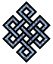fail2ban bad ip database: ip 191.232.214.124

| ip database | live view | stats | report | help | api key:

 ip: 191.232.214.124 hostname: 191.232.214.124 country:[BR] Brazil first reported: 17.11.2018 00:28.04 GMT+0200 last reported: 06.02.2019 13:27.35 GMT+0200 time period: 81d 12h 59m 31s total reports: 11 reported by: 3 host(s) filter(s): ssh (7) ssh (4) tor exit node no badips.com db Lookupport scan of '191.232.214.124':

[-hide]
```# Nmap 6.40 scan initiated Sat Nov 17 00:29:01 2018 as: /usr/bin/nmap -sU -sS -O 191.232.214.124
Nmap scan report for 191.232.214.124
Host is up (0.19s latency).
Not shown: 1000 open|filtered ports, 994 filtered ports
PORT     STATE  SERVICE
22/tcp   open   ssh
80/tcp   open   http
81/tcp   closed hosts2-ns
443/tcp  open   https
3306/tcp open   mysql
8080/tcp closed http-proxy
No exact OS matches for host (If you know what OS is running on it, see http://nmap.org/submit/ ).
TCP/IP fingerprint:
OS:SCAN(V=6.40%E=4%D=11/17%OT=22%CT=81%CU=%PV=N%G=Y%TM=5BEF52ED%P=x86_64-pc
OS:-linux-gnu)SEQ(SP=106%GCD=1%ISR=10C%TI=Z%TS=A)OPS(O1=M5A0ST11NW7%O2=M5A0
OS:ST11NW7%O3=M5A0NNT11NW7%O4=M5A0ST11NW7%O5=M5A0ST11NW7%O6=M5A0ST11)WIN(W1
OS:=7120%W2=7120%W3=7120%W4=7120%W5=7120%W6=7120)ECN(R=Y%DF=Y%TG=40%W=7210%
OS:O=M5A0NNSNW7%CC=Y%Q=)T1(R=Y%DF=Y%TG=40%S=O%A=S+%F=AS%RD=0%Q=)T2(R=N)T3(R
OS:=N)T4(R=N)T5(R=Y%DF=Y%TG=40%W=0%S=Z%A=S+%F=AR%O=%RD=0%Q=)T6(R=N)T7(R=N)U
OS:1(R=N)IE(R=N)

OS detection performed. Please report any incorrect results at http://nmap.org/submit/ .
# Nmap done at Sat Nov 17 00:29:49 2018 -- 1 IP address (1 host up) scanned in 48.31 seconds
```
```Σ = 60 | Δt = 0.0050599575042725s
```